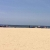# Erlang排列组合示例中引发对列表解析的一些疑问

crispdog 发布于 2015/12/14 13:13

#### 问题出现在书（pdf）中一段用 "列表解析" 进行 "排列组合" 的代码：

```perms([]) -> [[]];
perms(L) -> [[H|T] || H <- L, T <- perms(L--[H])].```

#### 按照我对列表的初级认识：

`[1,2] = [1 | ].`
` [1 | ] = [1 | [2 | [] ]].`

#### 一步步看下:

```%% 我们只看第一条执行线路，其他先忽略
1  perms("12") -> [[1 | perms()]].
2  perms("2") -> [[ 2 | perms([])]].
3  perms([]) -> [[]].```

### 请高手指点迷津，不胜感激！

0perms([]) -> [[]];

perms(L) -> [[H|T] || H <- L, T <- perms(L--[H])].